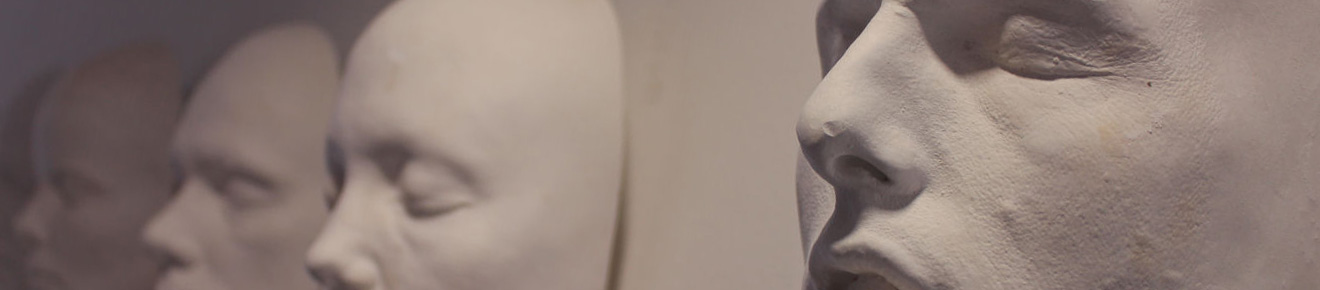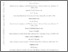# HST FUV spectroscopy of the short orbital period recurrent nova CI Aql: Implications for white dwarf mass evolution

Sion, EM, Wilson, RE, Godon, P, Starrfield, S, Williams, RE and Darnley, MJ (2019) HST FUV spectroscopy of the short orbital period recurrent nova CI Aql: Implications for white dwarf mass evolution. Astrophysical Journal, 872 (1). ISSN 0004-637XPreview
Text
HST FUV spectroscopy of the short orbital period recurrent nova CI Aql.pdf - Accepted Version

An HST COS Far UV spectrum (1170 A to 1800 A) was obtained for the short orbital period recurrent novae (T Pyxidis subclass), CI Aquilae. CI Aql is the only classical CV known to have two eclipses of sensible depth per orbit cycle and also have pre- and post-outburst light curves that are steady enough to allow estimates of mass and orbital period changes. Our FUV spectral analysis with model accretion disks and NLTE high gravity photospheres, together with the Gaia parallax, reveal CI Aql's FUV light is dominated by an optically thick accretion disk with an accretion rate of the order of $4\times 10^{-8}$ $M_{\odot}/yr$. Its database of light curves, radial velocity curves, and eclipse timings is among the best for any CV. Its orbit period ($P$), $dP/dt$, and reference time are re-derived via simultaneous analysis of the three data types, giving a dimensionless post-outburst $dP/dt$ of $-2.49\pm 0.95\times 10^{-10}$. Lack of information on loss of orbital to rotational angular momentum leads to some uncertainty in the translation of $dP/dt$ to white dwarf mass change rate, $dM_1/dt$, but within the modest range of $+4.8\times 10^{-8}$ to $+7.8\times 10^{-8}$ $M_{\odot} /yr$. The estimated white dwarf mass change through outburst for CI Aql, based on simple differencing of its pre- and post outburst orbit period, is unchanged from the previously published $+5.3 \times 10^{-6} M_{\odot}$. At the WD's estimated mass increase rate, it will terminate as a Type Ia supernova within 10 million years.View Item